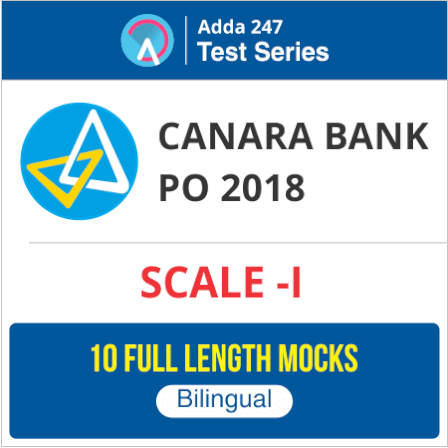### Reasoning Questions for IBPS Clerk 2018

Reasoning Ability is an onerous section. With the increasing complexity of questions, it becomes hard for one to give it the cold shoulder. The only way to make the grade in this particular section in the forthcoming banking exams like IBPS Clerk Prelims is to practice continuously with all your heart and soul. And, to let you practice with the best of the latest pattern questions, here is the Adda247 Reasoning Quiz based on the exact same pattern of questions that are being asked in the exams.

Directions (1-5): Study the following information carefully and answer the questions given below:
Ten persons Q, R, S, T, U, V, W, X, Y and Z are sitting in two rows with five persons in each row in such a way that one person in the first row sits exactly opposite and facing a person in the second row. Members of the first row are facing North.
R sits in the first row to the immediate right of X who sits exactly opposite of T. S is at the extreme end of the second row and is second to the left of T. Q is to the immediate right of T and exactly opposite of V. W sits exactly opposite to U who is at one of the ends of the second row. Z does not sit at the end.

Q1. Which of the following pairs of persons are sitting at the two ends of the first row?
(a) WZ
(b) UY
(c) WY
(d) UZ
(e) None of these

Q2. Who is second to the left of R?
(a) Y
(b) W
(c) X
(d) V
(e) None of these

Q3. Who is third to the left of U?
(a) T
(b) Y
(c) X
(d) S
(e) None of these

Q4. Q sits between which of the following persons?
(a) TZ
(b) UT
(c) VR
(d) RY
(e) None of these

Q5. Who sits exactly opposite of R?
(a) Z
(b) Y
(c) W
(d) Q
(e) None of these

Directions ( 6-8): Following questions are based on the information provided below.
‘A × B’ means ‘A is mother of B’.
‘A – B’ means ‘A is brother of B’.
‘A + B’ means ‘A is sister of B’.
‘A ÷ B’ means ‘A is father of B’.

Q6. Which of the following means ‘R’ is maternal uncle of ‘T’?
(a) R – M × T
(b) R + M × T
(c) T × M – R
(d) T + M ÷ R
(e) None of these

Q7. Which of the following means ‘F’ is paternal grandfather of ‘H’?
(a) F – J ÷ H
(b) F ÷ J – H
(c) F ÷ J ÷ H
(d) H ÷ J ÷ F
(e) None of these

Q8. How is K related to M in R ÷ M – K?
(a) Son
(b) Daughter
(c) Nephew
(d) cannot be determined
(e) None of these

Q9. How many pairs of letters are there in the word (in forward direction) APPLICATION, each of which have as many letters between then in the word as they have between then in the English alphabet?

(a) One
(b) Two
(c) Three
(d) Four
(e) None of these.

Q10. In a certain coding system, PAPER is written as PERPA and SUBJECT is written as JECTSUB, what should be the code for COUNCIL?
(a) NCILCOU
(b) LICNOUC
(c) NCOUCIL
(d) NLICUOC
(e) none of these

Directions (11-15): These questions are based on the following arrangement. Study it carefully and answer the questions.

Q11. Which element is sixth to the right of fifteenth from the right end?
(a) Q
(b) ¬
(c) L
(d) 1
(e) None of these

Q12. Four of the following five are alike in a certain way based on their positions in the above arrangement and so form a group. Which is the one that does not belong to the group?
(a) I#
(b) EQ
(c) 2¬
(d) \$2
(e) 5K

Q13. How many such symbols are in the above arrangement each of which is immediately followed by a number but not immediately preceded by another number?

(a) None
(b) One
(c) Two
(d) Three
(e) Four

Q14. If all the symbols are dropped from the above arrangement which element will be ninth from the left end?

(a) B
(b) 6
(c) 4
(d) I
(e) None of these

Q15. What will come in place of the question mark in the following series based on the above arrangement?

7T3 4#A BI6  ?
(a) R@I
(b) IQR
(c) R1@
(d) Q¬I

(e) None of these

You may also like to Read: